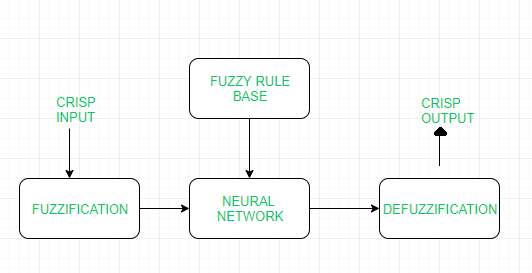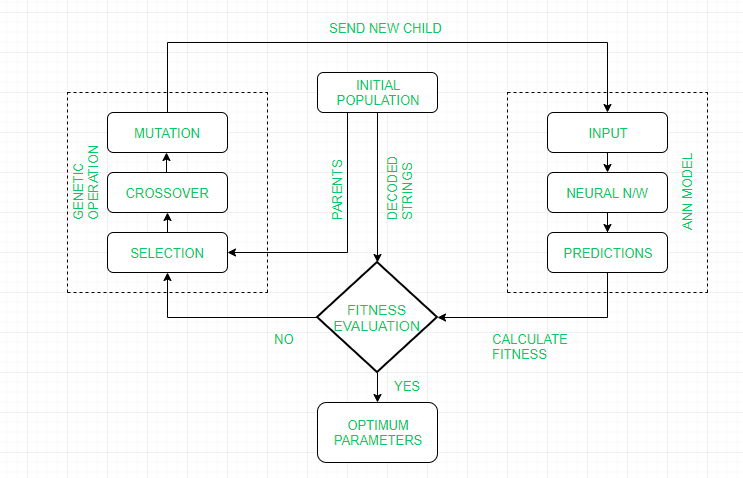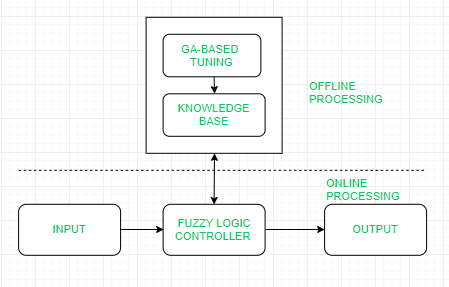# Introduction to ANN (Artificial Neural Networks) | Set 3 (Hybrid Systems)

• Difficulty Level : Medium
• Last Updated : 13 Oct, 2021

Prerequisites: Genetic algorithms, Artificial Neural Networks, Fuzzy Logic

Hybrid systems: A Hybrid system is an intelligent system that is framed by combining at least two intelligent technologies like Fuzzy Logic, Neural networks, Genetic algorithms, reinforcement learning, etc. The combination of different techniques in one computational model makes these systems possess an extended range of capabilities. These systems are capable of reasoning and learning in an uncertain and imprecise environment. These systems can provide human-like expertise like domain knowledge, adaptation in noisy environments, etc.

Types of Hybrid Systems:

• Neuro-Fuzzy Hybrid systems
• Neuro Genetic Hybrid systems
• Fuzzy Genetic Hybrid systems

(A) Neuro-Fuzzy Hybrid systems:

The Neuro-fuzzy system is based on fuzzy system which is trained on the basis of the working of neural network theory. The learning process operates only on the local information and causes only local changes in the underlying fuzzy system. A neuro-fuzzy system can be seen as a 3-layer feedforward neural network. The first layer represents input variables, the middle (hidden) layer represents fuzzy rules and the third layer represents output variables. Fuzzy sets are encoded as connection weights within the layers of the network, which provides functionality in processing and training the model.Working flow

• In the input layer, each neuron transmits external crisp signals directly to the next layer.
• Each fuzzification neuron receives a crisp input and determines the degree to which the input belongs to the input fuzzy set.
• The fuzzy rule layer receives neurons that represent fuzzy sets.
• An output neuron combines all inputs using fuzzy operation UNION.
• Each defuzzification neuron represents the single output of the neuro-fuzzy system.

• It can handle numeric, linguistic, logic, etc kind of information.
• It can manage imprecise, partial, vague, or imperfect information.
• It can resolve conflicts by collaboration and aggregation.
• It has self-learning, self-organizing and self-tuning capabilities.
• It can mimic the human decision-making process.

• Hard to develop a model from a fuzzy system
• Problems of finding suitable membership values for fuzzy systems
• Neural networks cannot be used if training data is not available.

Applications:

• Student Modelling
• Medical systems
• Traffic control systems
• Forecasting and predictions

(B) Neuro Genetic Hybrid systems:
A Neuro Genetic hybrid system is a system that combines Neural networks: which are capable to learn various tasks from examples, classify objects and establish relations between them, and a Genetic algorithm: which serves important search and optimization techniques. Genetic algorithms can be used to improve the performance of Neural Networks and they can be used to decide the connection weights of the inputs. These algorithms can also be used for topology selection and training networks.Working Flow:

• GA repeatedly modifies a population of individual solutions. GA uses three main types of rules at each step to create the next generation from the current population:
1. Selection to select the individuals, called parents, that contribute to the population at the next generation
2. Crossover to combine two parents to form children for the next generation
3. Mutation to apply random changes to individual parents in order to form children
• GA then sends the new child generation to ANN model as a new input parameter.
• Finally, calculating the fitness by the developed ANN model is performed.

• GA is used for topology optimization i.e to select the number of hidden layers, number of hidden nodes, and interconnection pattern for ANN.
• In GAs, the learning of ANN is formulated as a weight optimization problem, usually using the inverse mean squared error as a fitness measure.
• Control parameters such as learning rate, momentum rate, tolerance level, etc are also optimized using GA.
• It can mimic the human decision-making process.

• Highly complex system.
• The accuracy of the system is dependent on the initial population.
• Maintenance costs are very high.

Applications:

• Face recognition
• DNA matching
• Animal and human research
• Behavioral system

(C) Fuzzy Genetic Hybrid systems:
A Fuzzy Genetic Hybrid System is developed to use fuzzy logic-based techniques for improving and modeling Genetic algorithms and vice-versa. Genetic algorithm has proved to be a robust and efficient tool to perform tasks like generation of the fuzzy rule base, generation of membership function, etc.
Three approaches that can be used to develop such a system are:

• Michigan Approach
• Pittsburgh Approach
• IRL ApproachWorking Flow:

• Start with an initial population of solutions that represent the first generation.
• Feed each chromosome from the population into the Fuzzy logic controller and compute performance index.
• Create a new generation using evolution operators till some condition is met.

• GAs are used to develop the best set of rules to be used by a fuzzy inference engine
• GAs are used to optimize the choice of membership functions.
• A Fuzzy GA is a directed random search over all discrete fuzzy subsets.
• It can mimic the human decision-making process.

• Interpretation of results is difficult.
• Difficult to build membership values and rules.
• Takes lots of time to converge.

Applications:

• Mechanical Engineering
• Electrical Engine
• Artificial Intelligence
• Economics
My Personal Notes arrow_drop_up